# Python map: The Complete Guide

0
35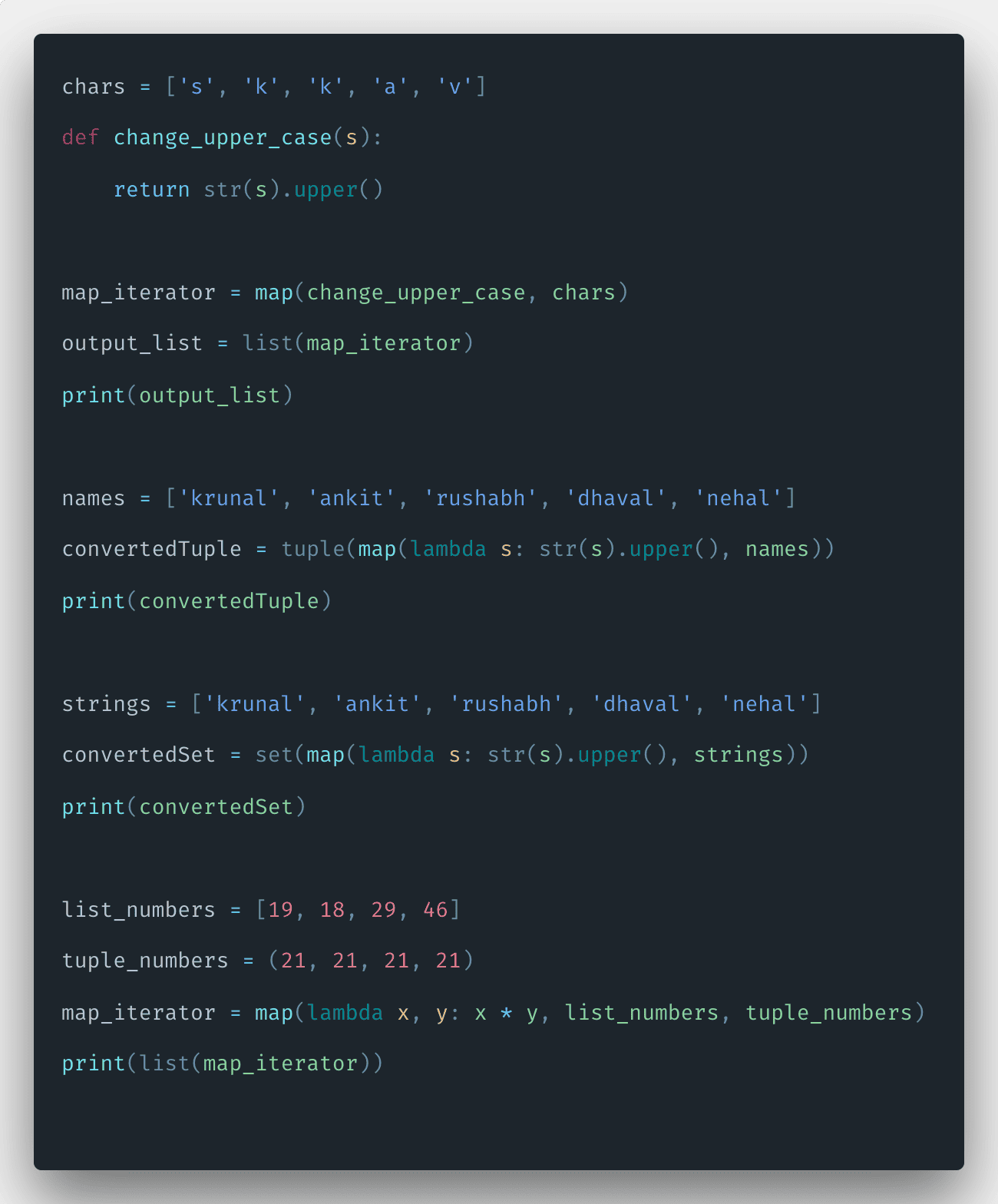Python map object is an iterator so that we can iterate over its elements. The map() function is a higher-order function taking a function and the variable number of iterables as arguments. The map() function supplied to the map will thereby be applied columnwise to the iterables.

## Python map

Python map() is a built-in function used to apply the function on all the elements of specified iterable and return map objects. The map() function applies the given function to each item of an iterable like the list, tuple, and returns the output as a list.

Python borrows the concept of the map from the functional programming domain. Map operation takes the mapping function and a vector of data as arguments. It returns the new vector, which is the outcome of applying the mapping function on each item of the vector independently.

### Syntax

The syntax of the map() is the following.

`map(function, iterable, ...)`

### Parameters

function – The map() method passes each item of the iterable to this function.

iterable –  An iterable which is to be mapped like a list, tuple, dictionary, or set.

Python map() function applies a given function to each item of an iterable and returns a list of the results.

### Return Value

The returned value from map() (map object) then can be passed to functions like list() (to create a list), set() (to create a set) and so on.

## How map() function works in Python

Okay, let’s take the following example.

```#app.py

def calculateCube(n):
return n*n*n

numbers = (11, 21, 19, 46)
output = map(calculateCube, numbers)
print(output)

# converting map object to set
numbersCube = set(output)
print(numbersCube)
```

See the output.

```➜  pyt python3 app.py
<map object at 0x102b5e4e0>
{97336, 1331, 9261, 6859}
➜  pyt```

In the above example, each item of the tuple is cubed.

Since map() expects the function to be passed in, lambda functions are commonly used while working with map() functions.

See the second following example.

```# app.py

chars = ['s', 'k', 'k', 'a', 'v']
def change_upper_case(s):
return str(s).upper()

map_iterator = map(change_upper_case, chars)
output_list = list(map_iterator)
print(output_list)```

In the above code, we have defined one list and defined a function that returns the uppercase string.

Then call a map function and provide the two arguments. 1) change_upper_case and 2) chars

The output from the map function is an iterator, and then we have converted that iterator to a list and printed that list.

So, the map function has mapped all lowercase chars to uppercase chars. Unlike the filter function, it does not remove any value from the existing list.

See the below output.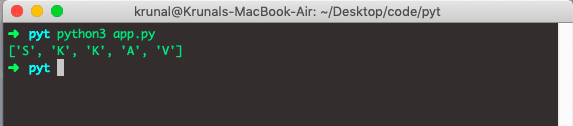## Python map() Function with Python Lambda Function

We can use the Python map() function with the Python Lambda function.

We can reduce the above code to three lines of code using the Lambda function.

We can use the lambda functions with a map() method if we don’t reuse it. For example, it is useful when our function is small and we don’t want to define a new function.

Let’s see the following example.

```# app.py

chars = ['s', 'k', 'k', 'a', 'v']
converted = list(map(lambda s: str(s).upper(), chars))
print(converted)```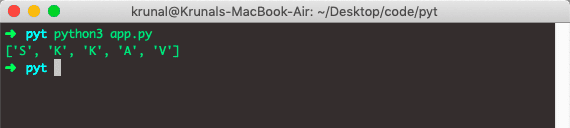## How to convert a Python map to the tuple and set

To convert a map to a tuple and set in Python, use the tuple() and set() method.

```# app.py

names = ['krunal', 'ankit', 'rushabh', 'dhaval', 'nehal']
convertedTuple = tuple(map(lambda s: str(s).upper(), names))
print(convertedTuple)```

See the below output.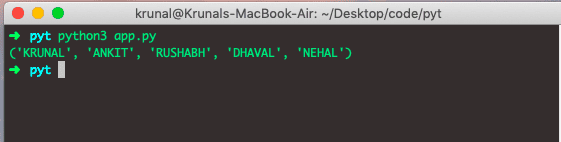We have converted the list to a tuple. Next, let’s convert the list to the set using a map and lambda function.

```# app.py

strings = ['krunal', 'ankit', 'rushabh', 'dhaval', 'nehal']
convertedSet = set(map(lambda s: str(s).upper(), strings))
print(convertedSet)```

See the below output.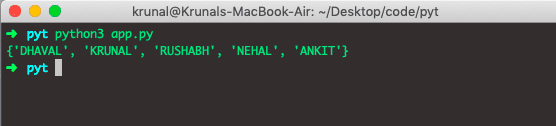## Python map() function with multiple arguments

The map() function can take multiple iterable arguments that return an iterator which you can convert into another list or set or dictionary.

Take a look at the example of using the map() function with multiple iterable arguments.

```# app.py

list_numbers = [19, 18, 29, 46]
tuple_numbers = (21, 21, 21, 21)
map_iterator = map(lambda x, y: x * y, list_numbers, tuple_numbers)
print(list(map_iterator))```

We have defined one list and iterator and passed that to the map function, and it will return the multiplication of that list and tuple and returns the iterator. We have converted that iterator to the list.

See the output.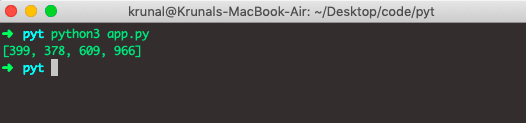## How to listify the list of strings individually in Python

To listify a list of strings in Python, use the map() function to create an iterator and then use the list() function to convert an iterator into the list.

```#app.py

listA = ['Millie', 'Finn', 'Noah', 'Gaten']
print("The original list is : " +  str(listA))

listifyData = list(map(list, listA))
print(listifyData)
```

See the output.

```➜  pyt python3 app.py
The original list is : ['Millie', 'Finn', 'Noah', 'Gaten']
[['M', 'i', 'l', 'l', 'i', 'e'], ['F', 'i', 'n', 'n'], ['N', 'o', 'a', 'h'], ['G', 'a', 't', 'e', 'n']]
➜  pyt
```

That’s it for this tutorial.